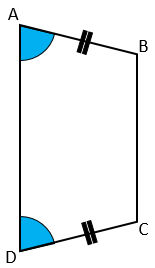# Exam-Style Questions on Proof

## Problems on Proof adapted from questions set in previous Mathematics exams.

### 1.

GCSE Higher

State whether each of the following statements is true or false. Give reasons for your answers.

(a) When $$a^2 = 25$$ the only value that $$a$$ can have is 5.

(b) When $$b$$ is a positive integer, the value of $$3b$$ is always a factor of the value of $$12b$$.

(c) When $$c$$ is positive, the value of $$c^2$$ is always greater than the value of $$c$$.

### 2.

GCSE Higher

One is added to the product of two consecutive positive even numbers. Show that the result is a square number.

### 3.

GCSE Higher

(a) Give a reason why 0 is an even number.

(b) The lengths of the sides of a right-angled triangle are all integers. Prove that if the lengths of the two shortest sides are even, then the length of the third side must also be even.### 4.

GCSE Higher

Betsy thinks that $$(3x)^2$$ is always greater than or equal to $$3x$$.

Is she is correct?

### 5.

GCSE Higher

Use algebra to prove that $$0.3\dot1\dot8 \times 0.\dot8$$ is equal to $$\frac{28}{99}$$.

### 6.

GCSE Higher

The diagram shows a quadrilateral ABCD in which angle DAB equals angle CDA and AB = CD.Prove that the diagonals of this quadrilateral are of equal length.

### 7.

GCSE Higher

m and n are positive whole numbers with m > n

m2 – n2 = (m + n)(m – n)

If m2 – n2 is a prime number prove that m and n are consecutive numbers.

### 8.

GCSE Higher

(a) Prove that the recurring decimal $$0.\dot2 \dot1$$ has the value $$\frac{7}{33}$$

(b) The value of $$x$$ is given as:

$$x=\frac{1}{5^{120}\times2^{123}}$$

Show that, when $$x$$ is written as a terminating decimal, there are 120 zeros after the decimal point.

(c) The reciprocal of any prime number $$p$$ (where $$p$$ is neither 2 nor 5) when written as a decimal, is always a recurring decimal.

A theorem in mathematics states:

The period of a recurring decimal is the least value of $$n$$ for which $$p$$ is a factor of $$10^n – 1$$

Marilou tests this theorem for the reciprocal of eleven.

She uses her calculator to show that 11 is a factor of $$10^2 – 1$$ then makes this statement:

"The period of the recurring decimal is 2 because 11 is a factor of $$10^2-1$$. This shows the theorem to be true in this case."

Explain why Marilou's statement is incomplete.

### 9.

GCSE Higher

$$\frac{p-1}{q-1}-\frac pq$$

Using your answer to part (a), prove that if $$p$$ and $$q$$ are positive integers and $$p \lt q$$, then

$$\frac{p-1}{q-1}-\frac pq\lt 0$$

### 10.

GCSE Higher

(a) Prove that the product of two consecutive whole numbers is always even.

(b) Prove, by giving a counter example, that the sum of four consecutive integers is not always divisible by 4.

The exam-style questions appearing on this site are based on those set in previous examinations (or sample assessment papers for future examinations) by the major examination boards. The wording, diagrams and figures used in these questions have been changed from the originals so that students can have fresh, relevant problem solving practice even if they have previously worked through the related exam paper.

The solutions to the questions on this website are only available to those who have a Transum Subscription.

Exam-Style Questions Main Page

Search for exam-style questions containing a particular word or phrase:

To search the entire Transum website use the search box in the grey area below.

Kausar Begum Khan, Kenya

Friday, January 26, 2018

"This is a fabulous website! I cannot thank you enough."

Tuesday, November 6, 2018

"Transum is so resourceful. The resources are so child-friendly that my students love its usage.It has a wide range of topics and caters to most of my needs. 'Thankyou" will be too small a word to express my gratitude to the content developers of this site."

Ahmed Jaja, Ghana

Thursday, November 22, 2018

"It was very useful for me.I recommend it to every math student.Thank you."

Tuesday, February 5, 2019

"A very great site.Nice questions."

Yuli, Woodmansterne

Tuesday, April 2, 2019

"This website is amazing it helps me with my maths to get good marks in my upcoming test. Thanks."

Do you have any comments? It is always useful to receive feedback and helps make this free resource even more useful for those learning Mathematics anywhere in the world. Click here to enter your comments.For All: